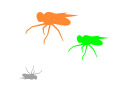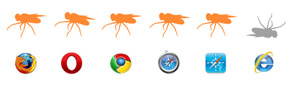# A JS App Under 1k: Evolving FliesJS1k.com ran a contest involving HTML5 Canvas and 1k of JavaScript. I decided to give it a try and learned a lot about JavaScript minification as well as some sneaky techniques I hope I don't repeat ;) Below is all about the app I created as well as some minification techniques I used to shrink my JS to a kilobyte.

## What does 1k of JavaScript look like?

```var w=500,j,k,M=Math,a=[],F="#ff88",v=document.body.childNodes[1
];v.width=v.height=w;c=v.getContext("2d");v.onmousedown=function
(A){j=A.clientX;k=A.clientY};for(i=0;i<16;)a[i++]=new z(p(32),p(
232),p(32),2*o(),16*o()+16);setInterval(function d(){g=c.createL
+96);c.fillStyle=g;c.fillRect(0,0,w,w);for(i in a){u=a[i];e=u.e;
y=u.y;x=u.x;if(!u.A||j>x&&j<x+e&&k>y&&k<y+e||u.a>u.q)u.A=0,u.y+=
9;else m(x,u.h,e),x=P,m(y,u.h,e),y=P;c.fillStyle="rgb("+u.r+","+
u.g+","+u.b+")";c.fillRect(x,y,e,e);if(y>w)f(),s=a[S],a[i]=new z
(p(s.r),p(s.g),p(s.b),l(s.h+n()*o()),l(s.e+n()*o()));u.a++}j=k=0
},32);function z(R,G,B,H,E){Q=this;Q.r=R;Q.g=G;Q.b=B;Q.h=H;Q.e=E
;Q.a=0;Q.q=999-E*H;Q.A=1;Q.x=o()*w;Q.y=o()*w;Q.s=E*9}function f(
){L=-255;for(I in a){U=a[I],Z=U.s+U.a*U.A;if(Z>L)S=I;L=Z}}functi
on p(A){A=~~l(A+n()*o()*32);return A>255?255:A}function m(A,B,C)
{P=l(A+n()*o()*B);P=P>w-C?w-C:P}function o(){return M.random()}f
unction n(){return o()>0.5?-1:1}function l(A){return M.abs(A)};```

## About the App

They live; they reproduce; they die. Sit back and watch them evolve based on the rules of their world, or take a more active role in their evolution by killing them. Kill the bigger flies, they evolve to be smaller. Kill the blue ones, they'll mutate to a different color. Try killing everything, they will tend to become harder to click (e.g. grow smaller, vibrate more, and colored like the background).

## Rules of Fly Town

• Bigger flies have a better chance of reproducing.
• Older flies have a better chance of reproducing.
• Use less energy, live longer.
• Vibrating requires energy.
• Size requires energy.

## Properties of a Fly

Vibration
Amount the fly wiggles around
Size
How big the fly is
Color
What color the fly is
Age
Current age in fractions of seconds
Longevity
Max age the fly can live
Aliveness
If the fly is alive or dead
Position
Where on the canvas the fly is

## Browser Compatibility

The contest requires browser compatiblity for: Firefox (3.6.8), Safari (5.0.1), Chrome (5.0.375.99), Opera (10.60). No Internet Explorer.View the demo

## The code (with comments and whitespace yay!)

Go Global: First we declare some global variables.

```var w = 500, // Canvas width and height
j, k, // Click x,y
M = Math, // Math Object
a = [], // Fly array
F = "#ff88";
```

HTML5 Canvas: Next we find the HTML5 canvas, assign its width and height, grab its context so we can draw all over it.

```// Do canvas things
v = document.body.childNodes; // Get canvas
v.width = v.height = w; // Set canvas dimensions
c = v.getContext("2d"); // Get context
```

User Input: If the user clicks on the canvas, save it and when the canvas gets redrawn we will do the dirty work. This will not handle page scrolling.

```// Click events
v.onmousedown = function(A) {
j = A.clientX; // Click X Position
k = A.clientY // Click Y Position
}```

Dawn of Time: Create the first flies, each slightly different (thats what those `o` and `p` functions do).

```// Create the first flies
for(i=0;i<16;) a[i++]=new z(p(32), p(232), p(32), 2*o(), 16*o()+16)```

Framerate: This function redraws everything we see. The `setInterval` calls the function at an interval of one fly year, because we will age the fly each time it is called. Each time the background and the flies are redrawn on the canvas. If a fly has been clicked it is killed.

```setInterval(
// Redraw function
function d() {
// Draw background gradient
g = c.createLinearGradient(0, 0, 0, w);
c.fillStyle = g;
c.fillRect(0, 0, w, w)
// For each fly
for(i in a) {
u = a[i];
e = u.e;
y = u.y;
x = u.x;

// Check if the fly died
if(!u.A || j > x && j < x + e && k > y && k < y + e || u.a > u.q)
u.A=0, // Mark fly as dead
u.y += 9 // Fly begins to drop
else m(x,u.h,e),x=P, // Move fly x position
m(y,u.h,e),y=P;// Move fly y position

// Draw fly
c.fillStyle = "rgb(" + u.r + "," + u.g + "," + u.b + ")";
c.fillRect(x, y, e, e) // Draw the fly

// The fly has dropped entirely off the canvas
if(y > w) // Delete fly
f(),
s = a[S], // Choose fly to reproduce
a[i] = new z(p(s.r), p(s.g), p(s.b), l(s.h + n() * o()), l(s.e + n() * o()));

u.a++ // Age the fly
}
j = k = 0 // Clear mouse click location
}
,32) // Redraw frequency aka one fly year (in milliseconds)```

Fly Reproduction: The `z` function creates a new fly. The `f` function decides which fly gets to reproduce.

```// ---------------------------- Fly Reproduction
// Create a new fly
function z(R, G, B, H, E) {
Q=this;
Q.r = R; // Red
Q.g = G; // Green
Q.b = B; // Blue
Q.h = H; // Vibration Amount
Q.e = E; // Size
Q.a = 0; // Age
Q.q = 999 - E*H; // Maximum Age (Vibration and Size take energy which shortens lifetime)
Q.A = 1; // Alive Boolean
Q.x = o() * w; // X Position
Q.y = o() * w; // Y Position
Q.s = E*9 // Macheesmo (probability of reproducing)
}
// Update a global index of the fly that gets to reproduce
function f() {
L=-255 // Largest macheesmo
for(I in a) { // For each fly
U=a[I],
Z=U.s + U.a*U.A // Calculate the probability of reproduction
if(Z > L) // If it is bigger than all the other flies
S=I; // Update the index of the lucky fly
L=Z // Update a 'local' variable
}
}
```

Mutate: The `p` function changes the color component by a random amount from zero to an eight. The `m` function returns a random position within the range of the canvas.

```// ---------------------------- Mutation
// Returns a color randomly changed
function p(A) {
A = ~~l(A + n() * o() * 32) // Move the color component randomly up or down
return A > 255 ? 255 : A // Ensure the color is with the rgb of range 0-255
}
// Updates a global to new random position within the boundries of the canvas
function m(A,B,C){
P = l(A + n() * o() * B); // Move position in a random amount (up to vibrate amount)
P = P > w - C ? w - C : P // Ensure position is on the canvas
}
```

Math: Create functions to save space!

```// ---------------------------- Math
// Return random number
function o() {
return M.random() // Return random decimal 0.0 to 1.0
}
// Returns positive or negative
function n() {
return o() > .5 ? -1 : 1 // Randomly return -1 or 1
}
// Return absolue value of parameter
function l(A) {
return M.abs(A) // Return the absolute value of parameter
}```

## Resources

The demo contest that started this
JS1k, 1k Javascript demo contest
@kuvos who is running the contest has a great list of minification techniques
Minification tricks
"Cowboy" Ben Alman has some great js minification techniques on his post describing his js1k entry
Organ1k, my JS1k contest entry
Dreaming In JavaScript provides insight into JavaScript bitwise operators
~, !, +, & -
Bitwise operators cover over at Mozilla Developer Center
Bitwise Operators
Javascript Minifier at Google Code
Closure Compiler
Yahoo!'s Minifier
YUI compressor